Next: Virial Analysis Up: Super- and Subsonic Flow Previous: Steady State Flow under   Contents

## Stellar Wind -- Parker Wind Theory

Stellar winds are observed around various type of stars. Early type (massive) stars have large luminosities; the photon absorbed by the bound-bound transition transfers its outward momentum to the gas. This line-driven mechanism seems to work around the early type stars. On the other hand, acceleration mechanism of less massive stars are thought to be related to the coronal activity or dust driven mechanism (dusts absorb the emission and obtain outward momentum from the emission).

Here, we will see the identical mechanism in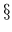2.8.1 and2.8.2 works to accelerate the wind from a star. Consider a steady state and ignore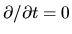. The continuity equation (2.1) gives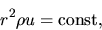(2.94)

where we used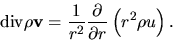(2.95)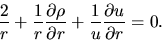(2.96)

The equation of motion (2.2) is as follows: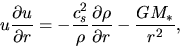(2.97)

where we used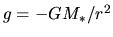(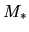means the mass of the central star). From these two equations (2.96) and (2.97) we obtain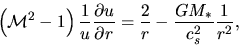(2.98)

where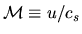represents the Mach number of the radial velocity. Take notice that this is similar to equations (2.89) and (2.93). That is, the fact that the rhs of equation (2.98) is positive corresponds to increasing the cross-section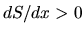. On the contrary, when the rhs is negative, the fluid acts as the cross-sectionis decreasing.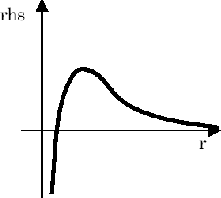For simplicity, we assume the gas is isothermal. The rhs of equation (2.98) varies shown in Figure 2.7. Therefore, near to the star, the flow acts as the cross-section of nozzle is decreasing and far from the star it does as the cross-section is increasing. This is the same situation that the gas flows through the Laval nozzle.

Using a normalized distance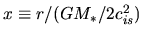, equation (2.98) becomes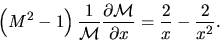(2.99)

This is rewritten as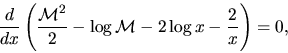(2.100)

we obtain the solution of equation (2.99) as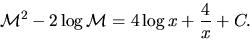(2.101)

This gives how the Mach number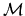varies changing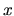. To explore this, we define two functions: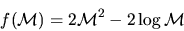(2.102)

is a function only depending onand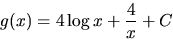(2.103)

is a function only depending on.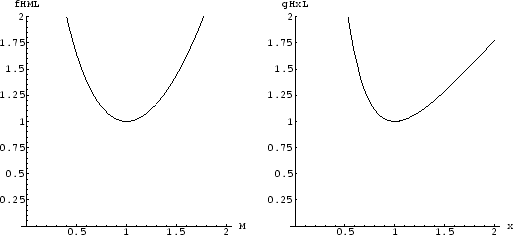Since the minima of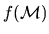and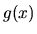are respectively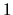and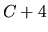, the permitted region in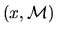changes for the values of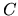.

1. If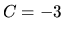, for all values of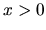there existwhich satisfies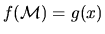. This corresponds to the two curves which pass through a critical X-point of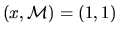in Figure 2.9.

2. If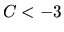, the minimum ofis smaller than that of. In this case, forwhere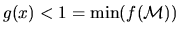, there is no solution. Thus,has solutions for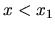and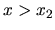, where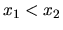, and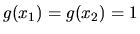. This corresponds to the curves running perpendicularly in Figure 2.9.

3. If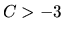, the minimum ofis smaller than that of. In this case,has solutions for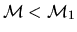and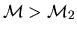, where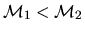, and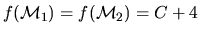. This corresponds to curves running horizontally in Figure 2.9.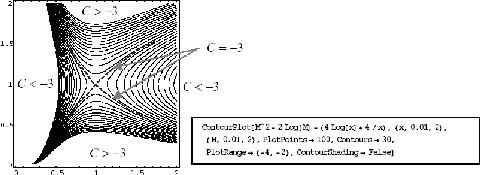Out of the two solutions of, a wind solution is one with increasingwhile departing from the star. This shows us the outflow speed is slow near the star but it is accelerated and a supersonic wind blows after passing the critical point. Since the equations are unchanged even if we replace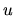with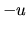, the above solution is valid for an accreting flow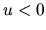. Considering such a flow, the solution running from the lower-right corner to the upper-left corner represents the accretion flow, in which the inflow velocity is accelerated reaching the star and finally accretes on the star surface with a supersonic speed.Next: Virial Analysis Up: Super- and Subsonic Flow Previous: Steady State Flow under   Contents
Kohji Tomisaka 2007-07-08